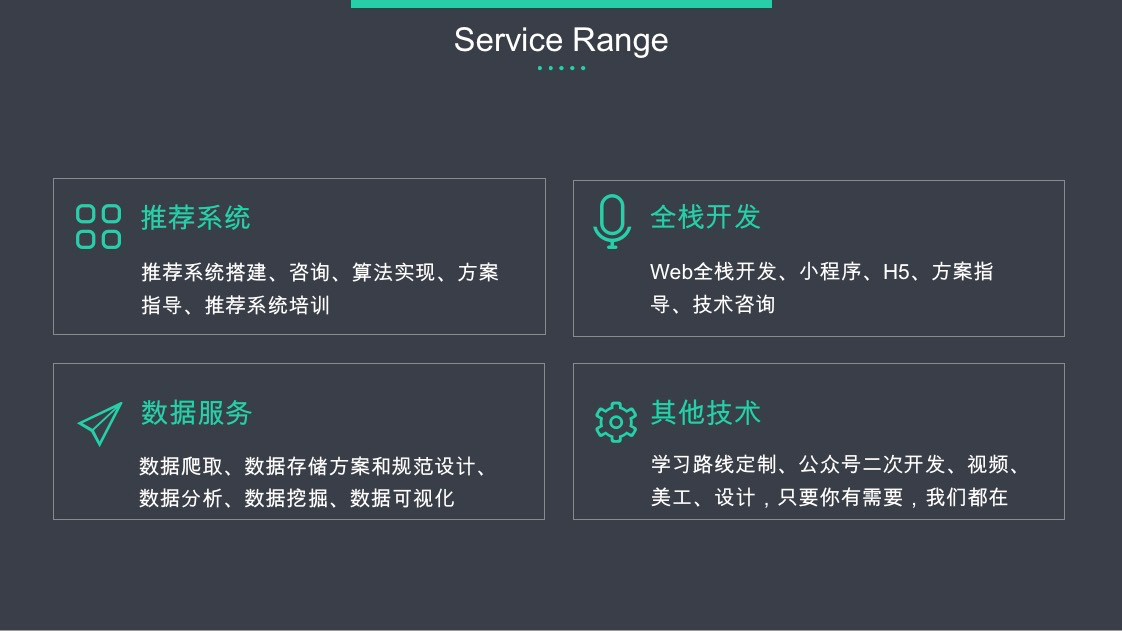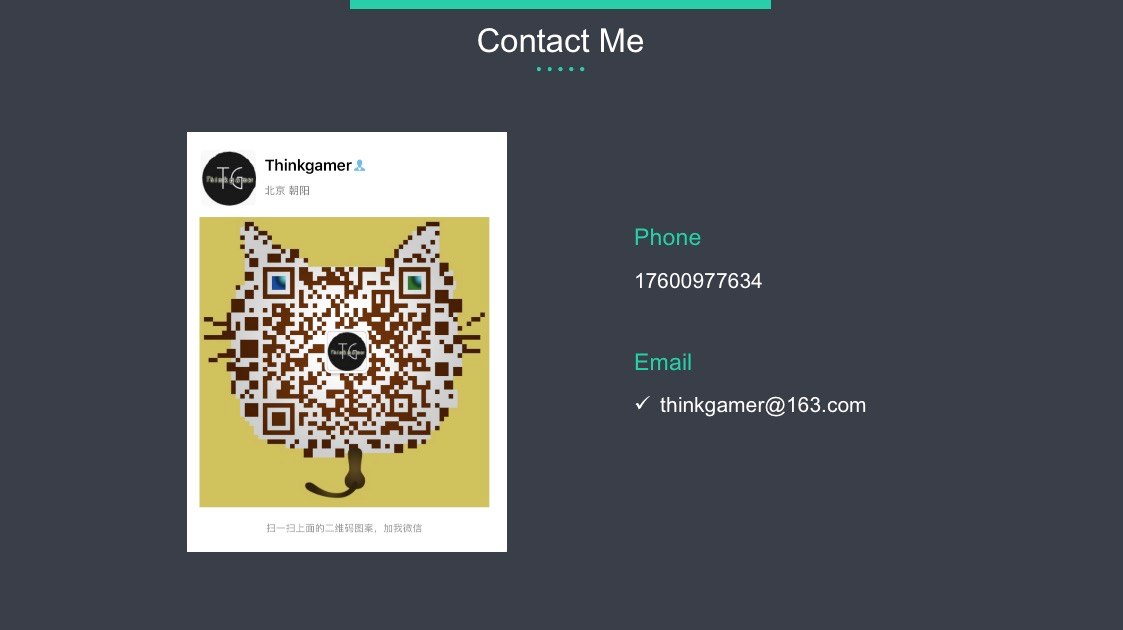# 1、聊一聊群组推荐

• 均值策略，即认为群组中每个人贡献是等价的，计算群组内top内容时，取对某内容贡献的均值。
• 最小化策略，即取群组内对某内容的最低贡献作为该内容的排序值
• 最大化策略，和最小化策略相反，即取群组内对某内容的最高贡献作为该内容的排序值
• 专家策略，根据用户在项目上的专业知识为其赋予了个性化的权重

AGREE的主要贡献点有：

• 第一次将神经网络中的注意力机制用于群组推荐，基于输入数据动态地学习融合策略。
• 引入用户和内容之间的交互行为，提升群组推荐的效果，同时在一定程度上能够缓解冷启动问题（首先根据某种策略得到群组，新用户来访时匹配其对应的群组，根据群组内其他成员选取的top内容作为新用户的冷启动内容）。
• 基于数据集（爬取的和公开的）进行了大量的实验，并进行公开，促进学术研究。

# 2、模型介绍

AGREE模型包含两部：

• （1）：基于小组成员聚集和偏好表示的群组表示学习
• （2）：通过NCF进行交互学习，为用户和群组推荐项目

## 2.1、符号表示含义和要解决的问题

• 粗体大写字母（eg：$\mathbf{X}$）表示矩阵
• 粗体小写字母（eg：$\mathbf{x}$）表示向量
• 粗体小写字母（eg：$x$）表示标量
• 花体字母（eg：$cal {X}$）表示集合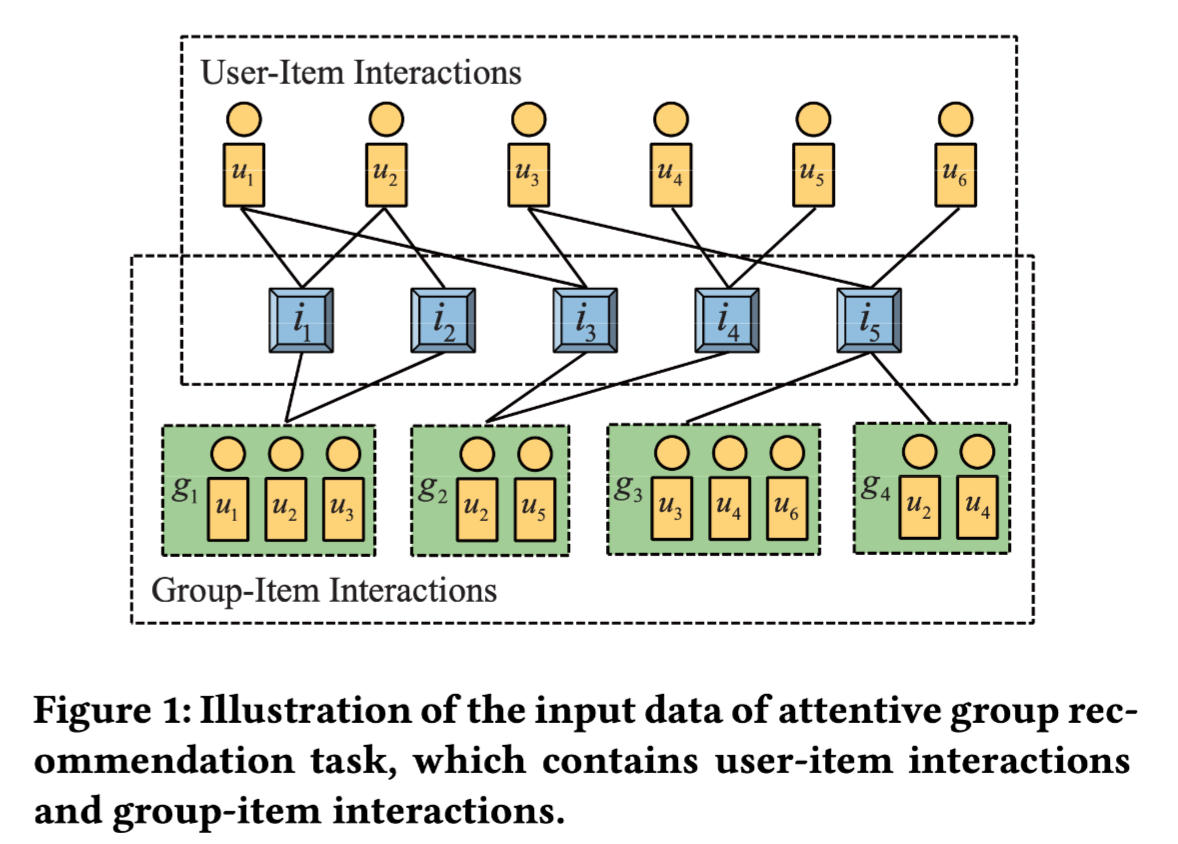• 输入是：用户集合$\{u_1, u_2, ….\}$，群组集合$\{g_1, g_2, g_3, …\}$，物品集合$\{v_1, v_2, v_3, …\}$，群组和物品的交互矩阵$Y$，用户和物品的交互矩阵$R$
• 输出是：两个个性化的排序函数，$f_g$（将一个物品映射给一个群组的分值）、$f_u$（将一个物品映射给一个用户的分值）

## 2.2、基于注意力的群组表征学习

$u_i$表示用户向量，$v_j$表示物品向量，我们的目标是通过群组成员的兴趣偏好为每个群组学习到一个向量，要从数据中动态学习聚合策略，可以将群组向量定义成依赖用户和物品的向量，抽象表示为：

$u_t$ 表示 $l$群组中的用户。$g_l(j)$表示群组$g_l$对物品$v_j$的偏好向量。在AGREE模型中，$g_l(j)$包括两部分：用户向量聚合和群组偏好向量。形式化表示为：

### 用户向量聚合

• $t \in g_l$表示群组$g_l$ 中用户的下标
• $P_v$ 和 $P_u$表示物品$v_j$和用户$u_t$对应的权重矩阵
• $b$ 表示偏置
• $ReLU(.)$ 表示激活函数
• $h^T$ 表示将结果映射为$o(j,t)$的权重向量
• $softmax(.)$ 表示将结果归一化的概率函数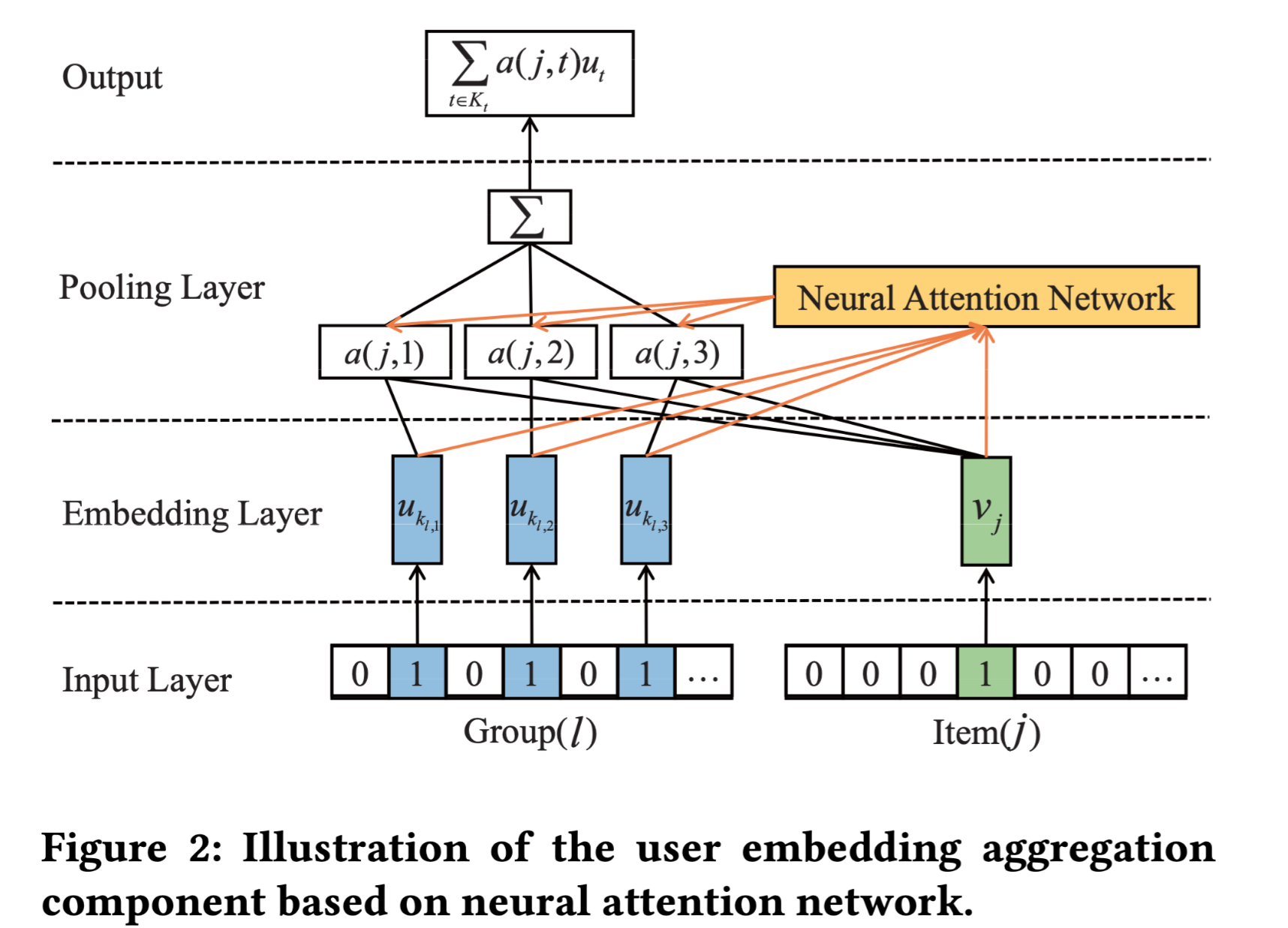## 2.3、基于NCF的交互学习

NCF是一个关于物品推荐的多层神经网络框架，可以参见之前的文章：Neural Collaborative Filtering。其主要思路是将用户和物品的向量，输入到一个定制的神经网络中，基于历史数据学校用户和物品之间的交互，由于神经网络具有很强的表达能力，NCF比传统的矩阵分解模型效果要好很多。因此我们选择了NCF框架对用户、物品的表达向量和用户-物品之间的交互进行端到端的学习。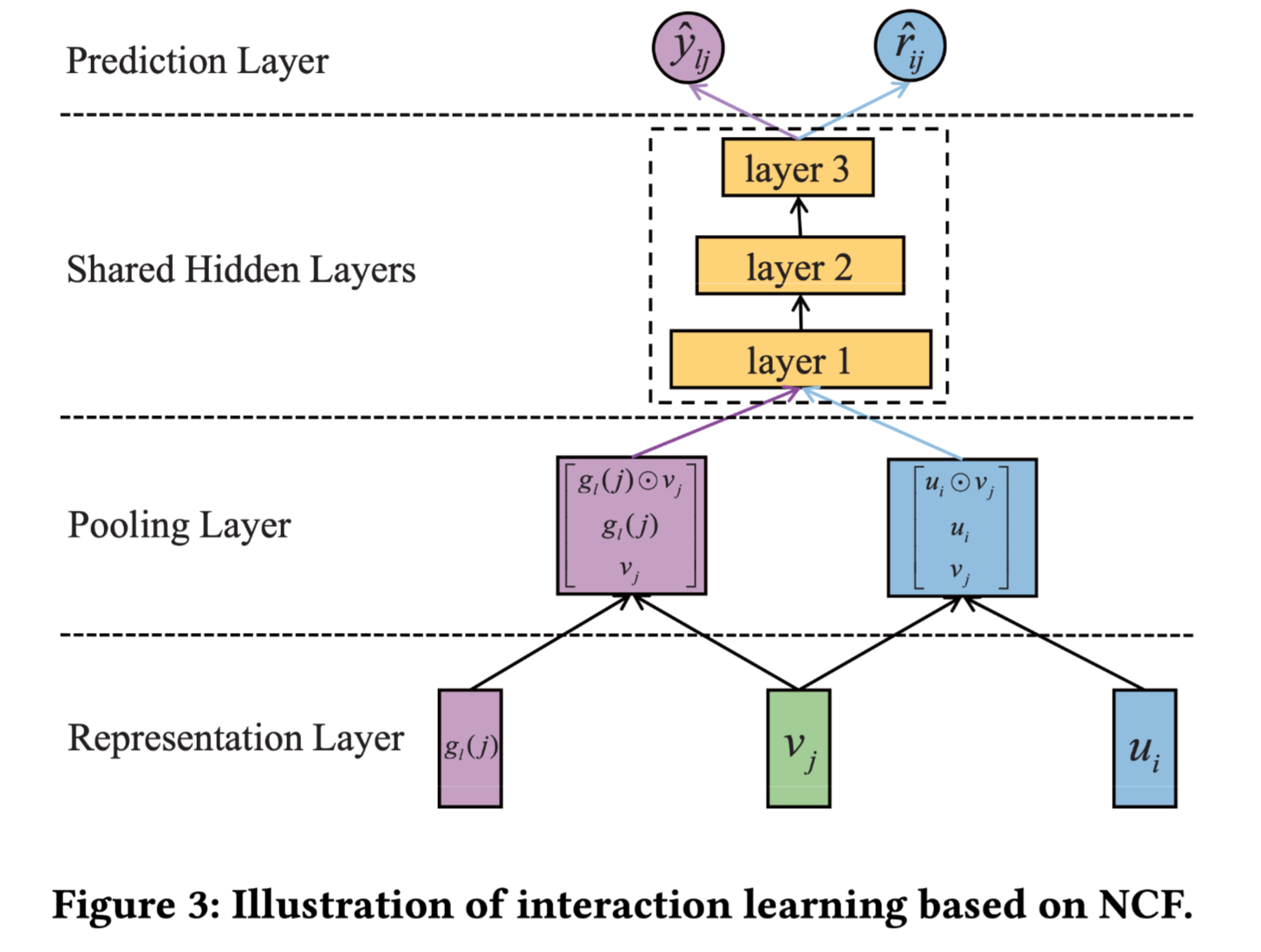（隐藏层），（hidden layer被两个任务进行共享），最后获得预测分数。

### hidden layer

• $W_h$ 表示第$h$层的权重矩阵
• $b_h$ 表示第$h$层的偏置
• $e_h$ 表示第$h$层的输出
• $ReLU$ 表示激活函数

• $w$ 表示预测层的权重
• $\hat{r}_{ij}$ 表示 用户对物品的预测分
• $\hat{y}_{ij}$ 表示 群组对物品的预测分

## 2.4、模型优化

### 目标函数

• $O$ 表示训练集
• $(i,j,s)$ 表示用户$u_i$ 对物品$v_j$有行为，但对物品$v_s$没有行为 （$v_s$ 是负采样产生）
• $\hat{r}_{i,j,s} = \hat{r}_{i,j} - \hat{r}_{i,s}$ 表示观察到的交互$(u_i, v_j)$和为观察到的交互$(u_i, v_s)$之间的差值分数

### 优化技巧

1、mini-batch。需要注意的首先需要将训练集进行shuffle，即混洗，保证数据之间是无序的。

3、droupout。由于神经网络的拟合能力强，为了提升模型的泛化能力，这里采用了深度学习中比较常见的Droupout机制，不仅在第一层中去除了$p %$，而且隐藏层也进行了剔除。另外需要注意的是在训练的时候需要进行dropout，但是在预测的时候不需要dropout。

# 3、实验效果

• 自己爬取和整理的马蜂窝数据
• CAMAa2011

• Hit Ratio（HR）
• Normalized Discounted Cumulative Gain (NDCG)

• 负采样率：4
• 嵌入层使用Glorot初始化权重
• 隐藏层使用均值为0，标准差为0.1的高斯分布初始化权重
• 基于梯度优化的算法使用Adma优化，mini-batch设置为[128, 256, 512, 1024]，学习率设置为[0.001, 0.005, 0.01, 0.05, 0.1]
• 隐藏成第一层的维度和嵌入层维度相同，为32
• 激活函数：ReLU
• 每组实验参数进行5次实验，选取平均值

• NCF
• Popularity（根据物品的流行度进行推荐）
• COM（基于概率的方法生成群组推荐）
• GREE（AGREE去除注意力机制）

AGREE和GREE的实验效果对比结果如下：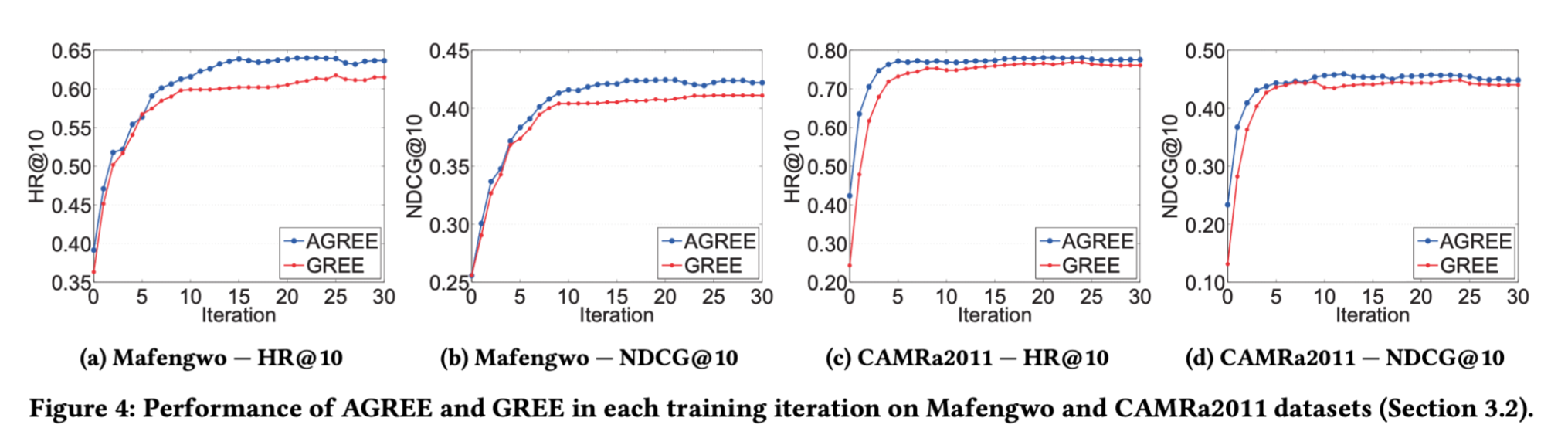• NCF+avg
• NCF+ the least misery strategy
• NCF + The maximum satisfaction strategy
• NCF + The expertise strategy

AGREE算法的有效性实验对比结果如下：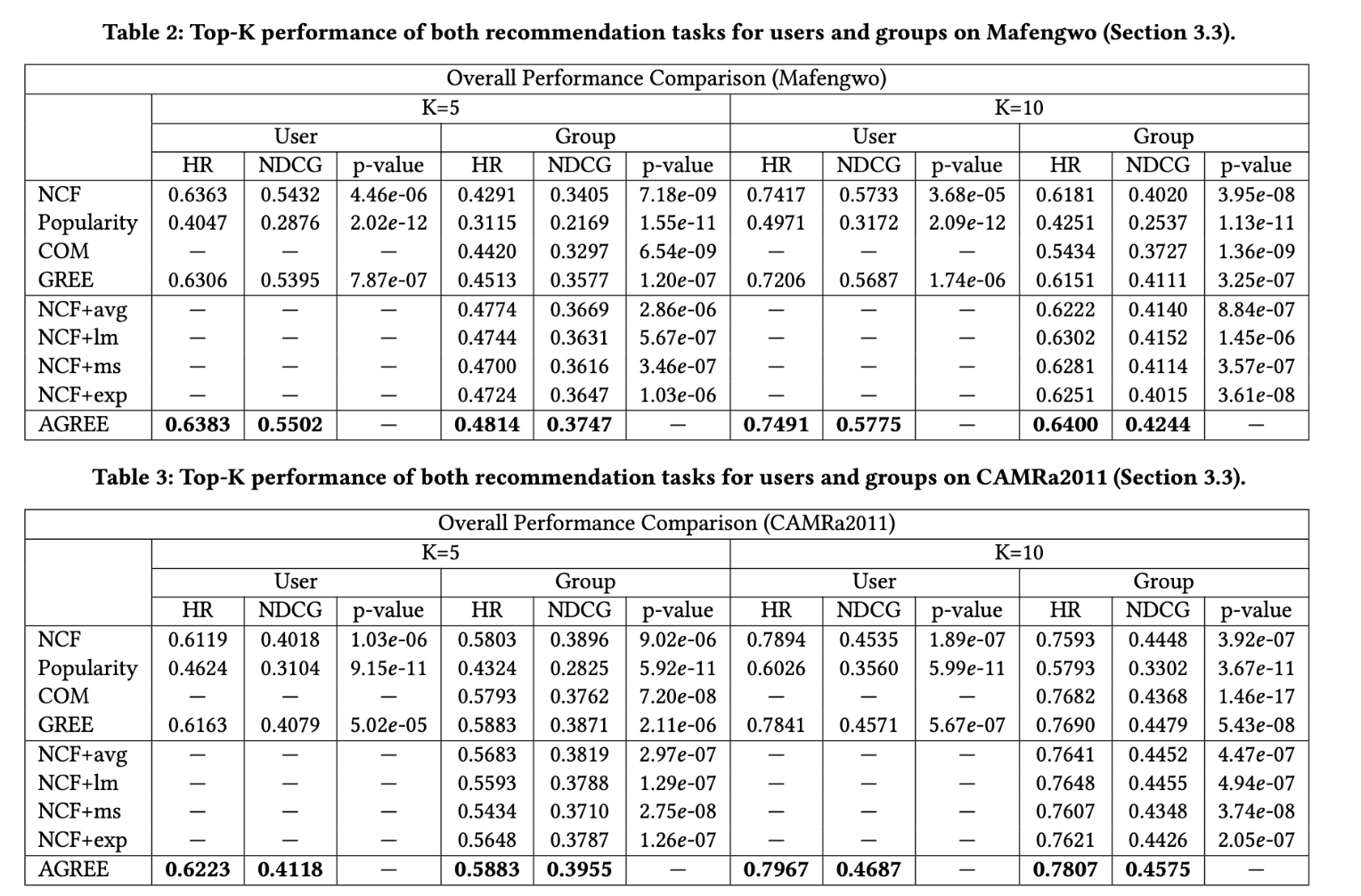• AGREE-U：AGREE算法中只利用了用户的embedding向量
• AGREE-G：AGREE算法中只利用了群组的embedding向量

AGREE算法和其变体的实验效果对比结果如下：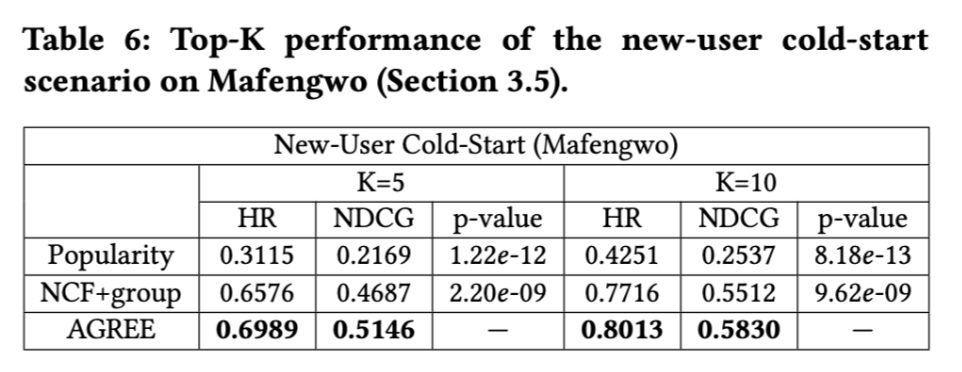# 4、代码解析

【技术服务】，详情点击查看： https://mp.weixin.qq.com/s/PtX9ukKRBmazAWARprGIAg